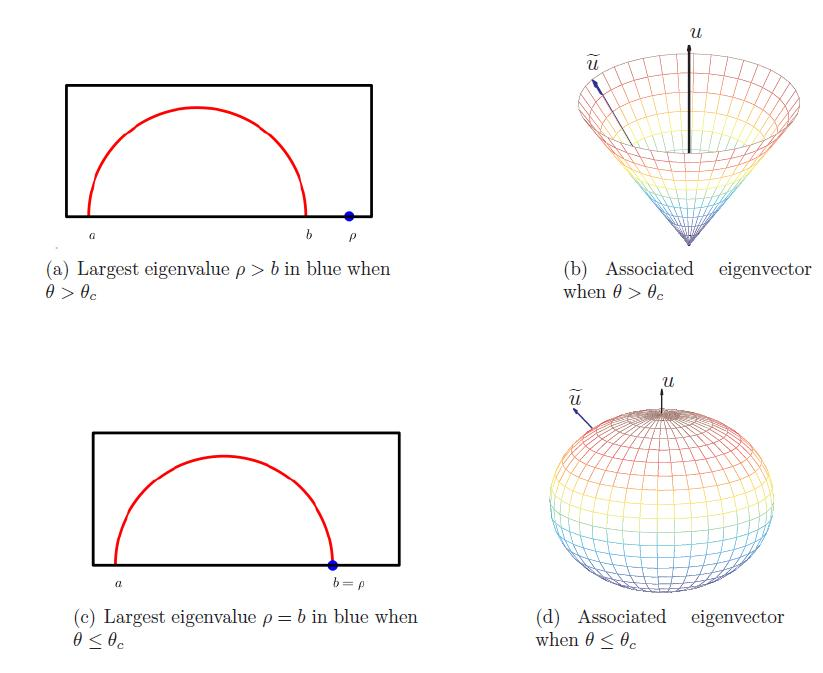## Bad News for PCAApril 20, 2010

Posted by Sarah in Uncategorized.
Tags: ,I just saw a talk by Raj Rao Nadakuditi about random matrix theory and the informational limit of eigen-analysis.

The issue is this. Lots of techniques for dealing with large data sets — PCA, SVD, signal detection — call for analyzing the eigenvectors and eigenvalues of a large matrix and reducing the dimensionality. But when do these friendly techniques fail us? It turns out that there is a phase transition, a signal-to-noise ratio below which we cannot distinguish the signal eigenvalues and eigenvectors from the noise. No matter how much data we collect, eigenvector-based dimensionality techniques will not distinguish signal from noise.

The simplest example Nadakuditi deals with is a Gaussian matrix perturbed by a rank-one matrix.is a Gaussian random matrix,is a normalized symmetric random matrix, andis the perturbed matrix, whereis a rank-one perturbation.

What is the largest eigenvalue? What about the corresponding eigenvector? Well, the Wigner semicircle law tells us that the noise eigenvalues follow a semicircular distribution, and the signal eigenvalue falls outside the semicircle. If you look at the top eigenvalue as a function of, there’s a critical value of 1 where the top eigenvalue begins to escape the semicircle. Below that critical value, there’s no way to distinguish the signal eigenvalue.

Peche and Feral developed a closed-form formula for the top eigenvalue:ifis greater than one, andotherwise.

But you can extend this to a broader range of situations. We can consider n x m matrices, and letandThis models a sample-covariance matrix. Again we have a phase transition:ifis greater thanandNadakuti’s theorem is in a more general case. If we assume we have a distribution of noise eigenvalues that converges, as the size of the matrix becomes large, to some continuous distributionlimited to the interval [a, b] thenifis less thanand b otherwise.

Here,refers to the Cauchy transformThe sketch of the proof is fairly simple.is an eigenvalue ofiff 1 is an eigenvalue ofEquivalently,Equivalently, the eigenvalues satisfy. Since theare assumed to be distributed uniformly on the sphere, this means thatSo the moral of the story is that we can determine, rather specifically, at what point a set of data is too noisy for dimensionality reduction techniques to have any hope of accuracy. There are analogues to this in the work on sparse approximation of Donoho and Tanner, and in free probability theory.

Edit: Here’s the link to the paper.